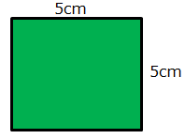Courses

# Worksheet Questions - Motion and Measurement of Distances Class 6 Notes | EduRev

## Science Class 6

Created by: Praveen Kumar

## Class 6 : Worksheet Questions - Motion and Measurement of Distances Class 6 Notes | EduRev

The document Worksheet Questions - Motion and Measurement of Distances Class 6 Notes | EduRev is a part of the Class 6 Course Science Class 6.
All you need of Class 6 at this link: Class 6

#### Q1. Fill in the blanks:

i. Measurement is the comparison of an unknown quantity with some              quantity.

Ans. fixed

ii. 1 km =            m.

Ans. 1000

iii. Standard unit of length is              .

Ans. metre

iv. The length of curved line can be measured using a               .

v.              is the distance between two points or ends of an object.

Ans. Length

vi.                   means the change in position of an object with time.

Ans. Motion

#### Q2. True/False:

i. The choice of device used for the measurement depends on the type of measurement to be made.

Ans. True

ii. Our senses are reliable for accurate measurement.

Ans. False

iii. 1m = 100cm.

Ans. True

iv. 5km = 5000cm.

Ans. False

v. Motion of a child on a swing is periodic motion.

Ans. True

vi. Motion of a wheel of a cycle is random motion.

Ans. False

#### Q3. Which measuring device can be used to measure the girth of a tree?

Ans. Measuring tape

#### Q4. Write the type of motion in which a body moves along a straight path.

Ans. Rectilinear or linear motion

Q5. Which unit of length is used to measure a large distance?

Ans. Kilometre

Q6. Write one example of circular motion.

Ans. Motion of hands of a clock

Q7. Write one example of rectilinear motion.

Ans. Motion of an apple falling from a tree

Q8. Write one example of periodic motion.

Ans. A bouncing ball

Q9. Name the type of motion in which a body moves along a curved path.

Ans. Circular or Rotational motion

Q10. Name the type of motion in which motion repeats after equal time interval.

Ans. Periodic motion

#### Q11. The distance between Ria’s house and temple is 1340 m. Express this distance in kilometre.

Ans. 1 km = 1000m

1340/1000 = 1.340 km

#### Q12. Find the length of square in mm.Ans. Each side = 5 x 10 = 50mm (All sides of square are equal)

#### Q13. Define ‘unit’.

Ans. The fixed quantity with which we compare an unknown quantity is called unit.

Q14. When object is said to be in rest?

Ans. If the position of an object does not change with time, it is said to be at rest.

Q15. What do you understand by ‘measurement’?

Ans. Measurement means the comparison of some unknown quantity with a known fixed quantity of same kind.

Q16. What is the common in between hand span, pace, angul and fist?

Ans. All of these are non-standard units of measurement that changes from person to person.

Q17. When object is said to be in motion?

Ans. When an object changes its position with time with respect to another object, it is said to be in motion.

Q18. Why is the hand span not considered as a reliable unit for measuring the length?

Ans. Hand span is not considered as a reliable unit for measuring the length because length of hand span varies from person to person.

Q19. What do you understand by standard unit of measurement?

Ans.The unit which is acceptable to the majority of people as a basic unit of measurement is called standard unit of measurement.

Offer running on EduRev: Apply code STAYHOME200 to get INR 200 off on our premium plan EduRev Infinity!

,

,

,

,

,

,

,

,

,

,

,

,

,

,

,

,

,

,

,

,

,

;